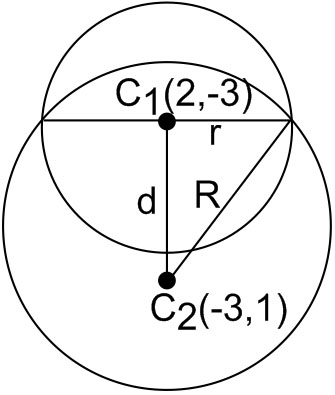#### If one of the diameters of the circle, given by the equation, x2 + y2 − 4x + 6y − 12=0, is a chord of a circle S, whose centre is at (−3, 2), then the radius of S is : Option 1)Option 2)Option 3) 5 Option 4) 10As we learnt in

General form of a circle -- wherein

centre =radius =Distance between centres=andNowOption 1)Incorrect option

Option 2)Correct option

Option 3)

5

Incorrect option

Option 4)

10

Incorrect option

#### divya.saini